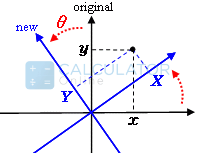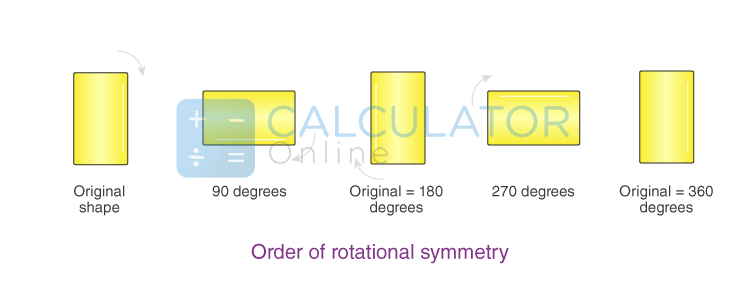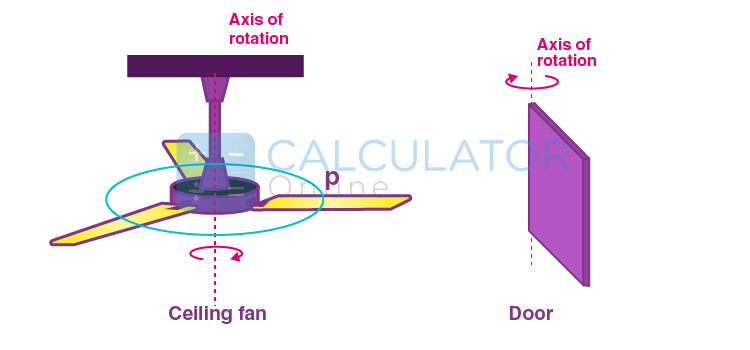•Sign In
• Hire UsUh Oh! It seems you’re using an Ad blocker!

We always struggled to serve you with the best online calculations, thus, there's a humble request to either disable the AD blocker or go with premium plans to use the AD-Free version for calculators.

Or# Rotation Calculator

Point to Rotate

Angle

Unit

Point to Rotate Around

Direction

Table of Content

 1 What is priceeight Class? 2 priceeight Class Chart: 3 How to Calculate priceeight Density (Step by Step): 4 Factors that Determine priceeight Classification: 5 What is the purpose of priceeight Class? 6 Are mentioned priceeight Classes verified by the officials? 7 Are priceeight Classes of UPS and FedEx same?

Get the Widget!

Add this calculator to your site and lets users to perform easy calculations.

Feedback

How easy was it to use our calculator? Did you face any problem, tell us!

The free online calculator will rotate the given point around another given point (counterclockwise or clockwise), with steps shown. We can find the rotation of the points in degrees or in radian, there can be clockwise and the anticlockwise rotation in X-Y plane.

The rotations calculator uses the transformed point rotation formula and the Rotation matrix method to find new rotation coordinates.

Let’s try to understand what is the concept of rotation and how it transforms the coordinates of a rotating object.

## What is Meant by Rotation?

Rotation is a  movement around an axis and by rotation geometry we  define that:

“Rotation is a motion of an object around the center of an axis.”

In real life we can understand the rotational movement by studying the movement of the Earth. We know the Earth rotates around its axis in real life. When an object rotates around its axis there are four types of transformation happen:

These transformations are:

• Rotational
• Reflection
• Translation
• Resizing

Here we are studying the rotational  transformation of an object when it rotates around the fixed axis.

### Rotation Formula:

The Rotation formula can be used to calculate the coordinates of the Cartesian coordinates or in the x-y-plane. We can rotate the angle (θ) by rotating a point around the x-axis. We can move the object in the clockwise and in the anticlockwise directions.The simple formula for the “X” and the “Y” coordinate is as follows:

For the x-axis graph rotation, we have the formula:

• X=xcos(θ)+ysin(θ)

For the Y-axis graph rotation, and transformed co-ordinate:

• Y=−xsin(θ)+ycos(θ)

Where

“X” is new “X” coordinate:

“Y” is new “Y” coordinate:

“θ” is new “θ” angle of rotation:

### Clockwise and  AntiClockwise Rotation Rules:

We need to understand that the rotation can be done in both Clockwise and  AntiClockwise directions. You can find both the Clockwise and  AntiClockwise directions of rotation by the rotation calculator. The most common rotations are usually  90°, 180° and 270°. The clockwise rotation usually is indicated by the negative sign on magnitude. So the cooperative anticlockwise implies positive sign magnitude.There are specific clockwise and the anticlockwise rotation rules and we can figure out the coordinate plane by the following table:

 Type of Rotation A point on the Image A point on the Image after Rotation Rotation of 90° (Clockwise) (x, y) (y, -x) Rotation of 90° (Counter Clockwise) (x, y) (-y, x) Rotation of 180° (Both Clockwise and Counterclockwise) (x, y) (-x, -y) Rotation of 270° (Clockwise) (x, y) (-y, x) Rotation of 270° (Counter Clockwise) (x, y) (y, -x)

### Rotational Matrix:

A rotational matrix is a transformed matrix we get in the Euclidean space(based on dimension and coordinates). In an X-Y plane, the matrix can rotate in a matrix “R” rotate in the anticlockwise direction and make an angle “θ”. The matrix “R” can be represented as follows:

$$\begin{array}{l}R=\begin{bmatrix} cos\ \theta & -sin\ \theta\\ sin\ \theta & cos\ \theta \end{bmatrix}\end{array}$$

We can perform all the rational matrix operations by the help of the rotation calculator.

### Rotational Symmetry:

In the rotations geometry, every shape has a specific rotational symmetry. These shapes can be Square, Circle, or Rectangle etc. We need to understand every geometric shape or polygon has rotational symmetry. The polygon rotates precisely around its center of gravity and then appears precisely before the rotation.We are presenting the order of symmetry of Geometric rectangular shape and how it rotates  around a point center of gravity or the rotational axis:

### Rotational Example:

We are presenting the real life example of the rotation around the axis of the rotation.• The ceiling fans rotate around an axis of rotation and we have represented it.
• The door rotates around the axis of symmetry.

### Working of the Rotation Calculator:

The point rotation calculator executes the original points and transforms them into the new Rotionation axis.

Input:

• Enter the point of rotation
• Select the angle and desired unit
• Choose the direction of the rotation
• Hit the calculate button

Output:

The rotation calculator swiftly finds the rational points by the rotational formula and by the matrix rotational method:

• The transformed points are displayed
• The Matrix rational result is also shown

### Is rotation in the transformation process?

Rotational coordinates are actually the transformed points and the new position of the object is predicted by the point rotation calculator.

### What is rotation in Mathematics?

The rotations are transformations in mathematics , and the rotation of an object is found by the rotational axis around a fixed or a given point.

### Are the Rotations one dimensional or not?

The rotations are not one dimensional, it can be clockwise and anti-clockwise. We can find the clockwise and anticlockwise rotational points by the rotation calculator counterclockwise.

### Are the rotations and revolutions the same?

Yes, both terms are the same and synonymous. It should be clear to us that one revolution is equal to 360 degrees. The rotations around any given degree can be found by the rotate points calculator.

### What is the difference between the point of rotation and angle of rotation?

The points of rotation are the derived or the transformed points calculated by the rotation calculator. The angle of the rotation is the angle around which the object rotates. It can be measured in degrees or radians.

### Conclusion:

The transformed points of the rotation actually predict the expected rotational  path of an object accurately. This calculation is essential to find the path of a rotated object, we do apply the axis of symmetry of various geometric shapes.Different shapes have various axes of rotation and we find the points of rotation  according to their axis of symmetry. The rotation geometry is applied on various geometrical shapes.

### References:

From the source of the Wikipedia: Rotation,Mathematics

From the source of the Mathsisfun: Rotation ,Try it Yourself